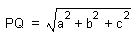HOME MATH DICTIONARY DOWNLOAD FEEDBACK DISCLAIMER
 Question: What is Substitution ? Answer: To substitute means to replace one thing by another. Substitution is the process of replacing the letters in a formula by numbers. For example, the surface area A cm2 of a cylinder of radius 5cm and height 12 cm can be calculated by substituting 5 for r and 12 for h in the formula A = 2 π r (r + h) A = 2 π x 5 x (5 + 12) = 170 π But substitution can also involve substituting one algebraic expression for another. For example, if a shoe box has dimensions a, b and c and it is required to find the length of the diagonal PQ, then by Pythagoras theorem PQ2 = PR2 + RQ2 But PR2 = a2 + b2 And RQ = c So substituting for PR and RQ PQ2 = a2 + b2 + c2 from which